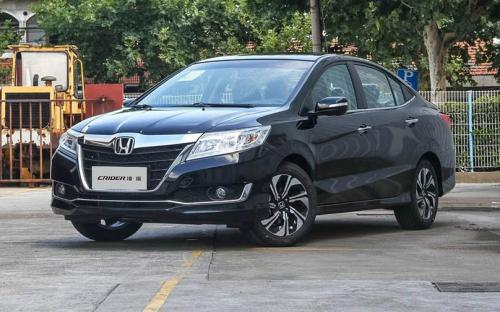### 广汽本田 凌派2017款最低售价：12.48 万元起

4664(mm)1750(mm)1505(mm)##### 配置亮点：
• 胎压监测装置

• ISOFIX儿童座椅接口

• 车身稳定控制(ESC/ESP/DSC等)

• 电动天窗

• 定速巡航

• 后倒车雷达

• 真皮座椅

• GPS导航系统

• 氙气大灯

• 后视镜加热

• 提交
2017款 1.8L CVT舒适特装版 (112张)
• 2017款 1.8L CVT舒适特装版 (112张)
• 2017款 1.8L CVT豪华特装版 (126张)
• 广汽本田 凌派 绕车实拍• 广汽本田 凌派 在售车型

排量 车型 厂商指导价 本地最低报价 购车工具
L
1.8L CVT舒适特装版 CVT无级变速
12.48万
12.48万

13.48万
13.48万

广汽本田 凌派 经销商

查看更多 >>

### 广汽本田 凌派 动力加速

凌派 0-100公里加速时间分布在 0.0--秒 属于 超跑级

动力级别 加速时间 车型

广汽本田 凌派 视频

广汽本田 凌派 新闻资讯

# 本田凌派特装版上市 售12.48-13.48万

上市新车 超过13280次关注

6月30日，广汽本田宣布凌派50万纪念特装版车型上市。新车共包含1.8LCVT舒适特装版和1.8LCVT豪华特装版两款车型，售价分别为12.48万元和13.48万元。

# 广汽本田召回621003辆雅阁/凌派车型

碰撞安全 超过13229次关注

日前，广汽本田汽车有限公司向国家质检总局备案了召回计划，决定自2017年9月25日起，召回部分雅阁及凌派车型，共计621003辆。

# 广汽本田新款凌派上市 售价10.98-14.98万

国产新车 超过7871次关注

广汽本田官方宣布，新款凌派车型正式上市，新车在外观上采用了本田最新的家族式设计风格，提供7种车身颜色供选；内饰基本与老款一致，依然提供深色和浅色两种，搭载...

# 广汽本田凌派底盘解析 更加注重细节

技术 超过7959次关注

凌派是广汽本田在今年推出的一款全新车型，厂家将其定位于紧凑型轿车，从它的车身数据来看也确实是一副紧凑型车的标准身材，然而很多人在看过凌派的外观之后却有一...

# 中国化定制 试驾评测广汽本田凌派

评测 超过4177次关注

一个运动化调校的底盘；一套由智能手机制造商开发的车载多媒体系统；一副随时能够接纳一家老小的家用车做派；凌派用自身最为鲜明的特点讲诉了本田品牌对中国年轻一...

# 13万高安全配置自动挡三厢车 广本凌派

导购 超过3939次关注

凌派的造型大胆而夸张，尤其是宽辐格栅的前脸，在这个级别车型中绝对是最独特的，虽然有被称为“中国龙”的设计元素拉近了与国人的距离，但看起来与中国普通消费者...

# C-NCAP碰撞 凌派1.8LAT 56.6分 获5星

碰撞安全 超过3866次关注

C-NCAP碰撞凌派1.8LAT56.6分获5星

# 凌派购车享4000元优惠 另赠装潢礼包

新闻 超过2802次关注

日前，编辑从广汽本田汽车恒明特约销售服务店了解到，目前凌派购车可享4000元优惠，另赠装潢礼包（贴膜、脚垫等），店内少量现车。感兴趣的朋友请致电经销商详询。

# 凌派现车销售 购车可优惠现金5000元

新闻 超过3660次关注

近日，编辑从巢湖伟安特约汽车销售服务有限公司了解到，目前店内凌派现车销售，购车可优惠现金5000元，对该车感兴趣的朋友可致电经销商详询。

# 购凌派优惠3000元现金 店内有现车销售

新闻 超过3942次关注

近日，编辑从广汽本田红山店获悉：店内凌派现车充足，购车优惠3000元现金，提供试乘试驾服务。

猜你喜欢

﻿
• 快速找车
• 选择品牌
• 选择品牌
• A  奥迪
• A  阿斯顿·马丁
• A  阿尔法·罗密欧
• B  宝沃
• B  布加迪
• B  巴博斯
• B  保时捷
• B  宾利
• B  奔驰
• B  宝马
• B  本田
• B  别克
• B  标致
• B  比亚迪
• B  宝骏
• B  北汽制造
• B  北汽新能源
• B  北汽幻速
• B  北汽威旺
• B  北京汽车
• B  奔腾
• B  北汽绅宝
• C  长安
• C  长安商用
• C  长城
• C  昌河
• D  大众
• D  道奇
• D  DS
• D  东南
• D  东风风神
• D  东风风行
• D  东风小康
• D  东风风度
• D  东风
• F  福特
• F  丰田
• F  菲亚特
• F  法拉利
• F  福田
• F  福迪
• F  福汽启腾
• G  观致
• G  广汽传祺
• G  广汽吉奥
• G  GMC
• H  红旗
• H  汉腾汽车
• H  哈弗
• H  哈飞
• H  海格
• H  海马
• H  华颂
• H  黄海
• H  华泰
• H  恒天
• J  吉利汽车
• J  捷豹
• J  Jeep
• J  江淮
• J  江铃
• J  金杯
• J  九龙
• J  金旅
• K  凯翼
• K  凯迪拉克
• K  克莱斯勒
• K  科尼塞克
• K  卡威
• K  开瑞
• L  路虎
• L  林肯
• L  劳斯莱斯
• L  兰博基尼
• L  雷克萨斯
• L  铃木
• L  雷诺
• L  理念
• L  力帆
• L  莲花汽车
• L  猎豹
• L  路特斯
• L  陆风
• M  马自达
• M  MG
• M  MINI
• M  玛莎拉蒂
• M  摩根
• M  迈凯轮
• N  纳智捷
• O  欧宝
• O  讴歌
• O  欧朗
• Q  奇瑞
• Q  起亚
• Q  启辰
• R  日产
• R  荣威
• R  瑞麒
• S  三菱
• S  斯威汽车
• S  萨博
• S  smart
• S  斯柯达
• S  斯巴鲁
• S  思铭
• S  双龙
• S  上汽大通
• S  双环
• T  特斯拉
• T  腾势
• W  沃尔沃
• W  五菱汽车
• W  五十铃
• W  威兹曼
• W  威麟
• X  现代
• X  雪佛兰
• X  雪铁龙
• X  西雅特
• Y  一汽
• Y  英菲尼迪
• Y  英致
• Y  依维柯
• Y  野马汽车
• Y  永源
• Z  众泰
• Z  中华
• Z  中兴
• Z  知豆
• 选择车系
• 选择车系
• 车型对比
• 选择品牌
• 选择品牌
• A  奥迪
• A  阿斯顿·马丁
• A  阿尔法·罗密欧
• B  宝沃
• B  布加迪
• B  巴博斯
• B  保时捷
• B  宾利
• B  奔驰
• B  宝马
• B  本田
• B  别克
• B  标致
• B  比亚迪
• B  宝骏
• B  北汽制造
• B  北汽新能源
• B  北汽幻速
• B  北汽威旺
• B  北京汽车
• B  奔腾
• B  北汽绅宝
• C  长安
• C  长安商用
• C  长城
• C  昌河
• D  大众
• D  道奇
• D  DS
• D  东南
• D  东风风神
• D  东风风行
• D  东风小康
• D  东风风度
• D  东风
• F  福特
• F  丰田
• F  菲亚特
• F  法拉利
• F  福田
• F  福迪
• F  福汽启腾
• G  观致
• G  广汽传祺
• G  广汽吉奥
• G  GMC
• H  红旗
• H  汉腾汽车
• H  哈弗
• H  哈飞
• H  海格
• H  海马
• H  华颂
• H  黄海
• H  华泰
• H  恒天
• J  吉利汽车
• J  捷豹
• J  Jeep
• J  江淮
• J  江铃
• J  金杯
• J  九龙
• J  金旅
• K  凯翼
• K  凯迪拉克
• K  克莱斯勒
• K  科尼塞克
• K  卡威
• K  开瑞
• L  路虎
• L  林肯
• L  劳斯莱斯
• L  兰博基尼
• L  雷克萨斯
• L  铃木
• L  雷诺
• L  理念
• L  力帆
• L  莲花汽车
• L  猎豹
• L  路特斯
• L  陆风
• M  马自达
• M  MG
• M  MINI
• M  玛莎拉蒂
• M  摩根
• M  迈凯轮
• N  纳智捷
• O  欧宝
• O  讴歌
• O  欧朗
• Q  奇瑞
• Q  起亚
• Q  启辰
• R  日产
• R  荣威
• R  瑞麒
• S  三菱
• S  斯威汽车
• S  萨博
• S  smart
• S  斯柯达
• S  斯巴鲁
• S  思铭
• S  双龙
• S  上汽大通
• S  双环
• T  特斯拉
• T  腾势
• W  沃尔沃
• W  五菱汽车
• W  五十铃
• W  威兹曼
• W  威麟
• X  现代
• X  雪佛兰
• X  雪铁龙
• X  西雅特
• Y  一汽
• Y  英菲尼迪
• Y  英致
• Y  依维柯
• Y  野马汽车
• Y  永源
• Z  众泰
• Z  中华
• Z  中兴
• Z  知豆
• 选择车系
• 选择车系
• 选择车型
• 选择车型
• 意见反馈